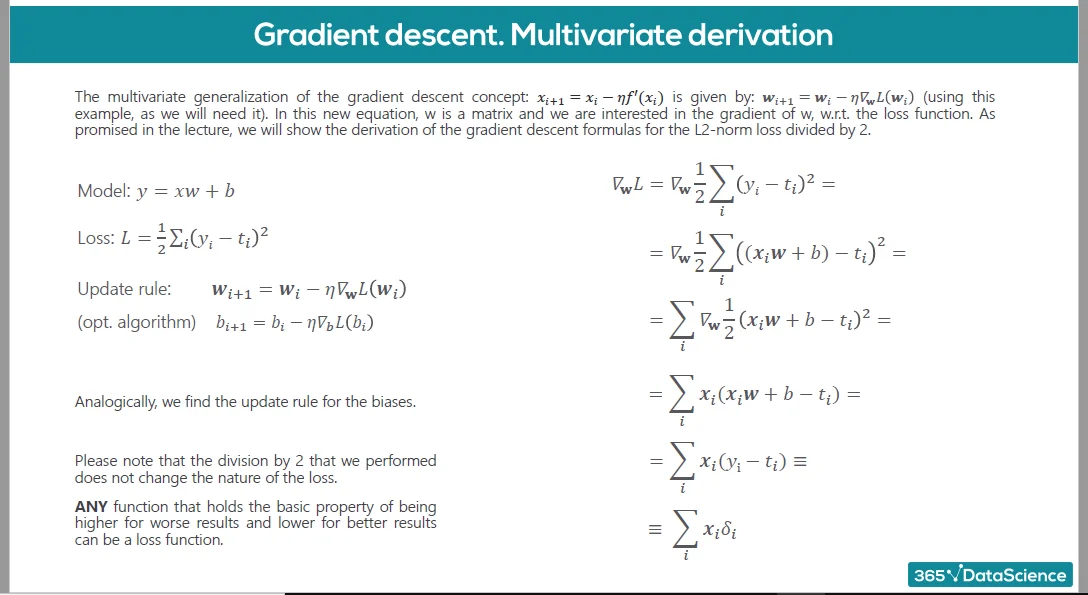Posted on:

03 Oct 2022

0

# More than one correct answer in the first question of the quiz + two other problems

Apart from the scripting error during all the lecture (x_i + 1 instead of x_(i+1)), I want to report that in question 1 you ask what the gradient descent deals with: it deals with many weights and biases, but it also deals with many inputs. So it doesn't make sense to say that the correct answer is just the first one and not the ladder.

Another thing: in this lecture you use y_i to indicate the i-th observation (with just 1 output for each observation), don't you? Previously, it was used to indicate the i-th output of a fixed observation if I well remember. I think it's a bit confusing.

And last thing:In the resources you show that y_i = x_i w + b, where y_i is a scalar (because I suppose that you consider just M=1 outputs), x_i is a vector (i-th row of the matrix x), w is a matrix (actually a vector because it's K x M = K x 1) and b is a scalar. But it should be b_i a scalar instead of b: if for example we have N=1000 observations, M=1 outputs and K=3 inputs, we have that y is an N x 1 = 1000 x 1 vector (then y_i is a scalar), x is an N x K = 1000 x 3 matrix (then x_i is a 1x3 vector), w is a K x M = 3 x 1 matrix and b is an N x 1 = 1000 x 1 vector (then b_i is a scalar). So I expect that y_25 = x_25 w + b_25 and the formula should have b_i instead of b.
Thanks